# Make your first Arduino robot - The best beginners guide!

This is a beginner's guide to making your first Arduino robot. Smart phone controlled, wall follower and obstacle avoiding robot.

BeginnerFull instructions provided1 hour80,247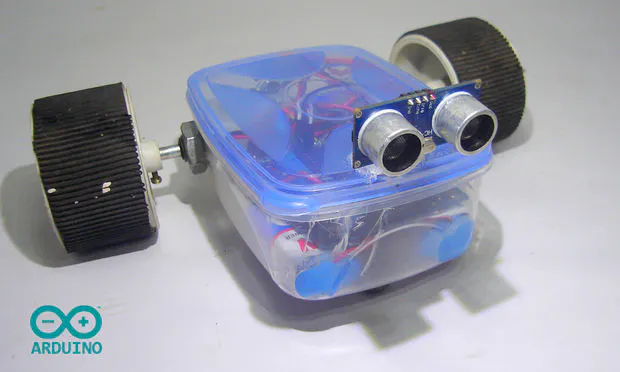## Things used in this project

### Hardware componentsArduino UNO & Genuino UNO
×1
 Adafruit L293D Motor Shield It's a low cost device, and it's easy to use. It's commonly available motor shield which does not require any previous skills. It can be also programmed easily using Adafruit library.
×1
 HC-SR4 Ultrasonic Sensor
×1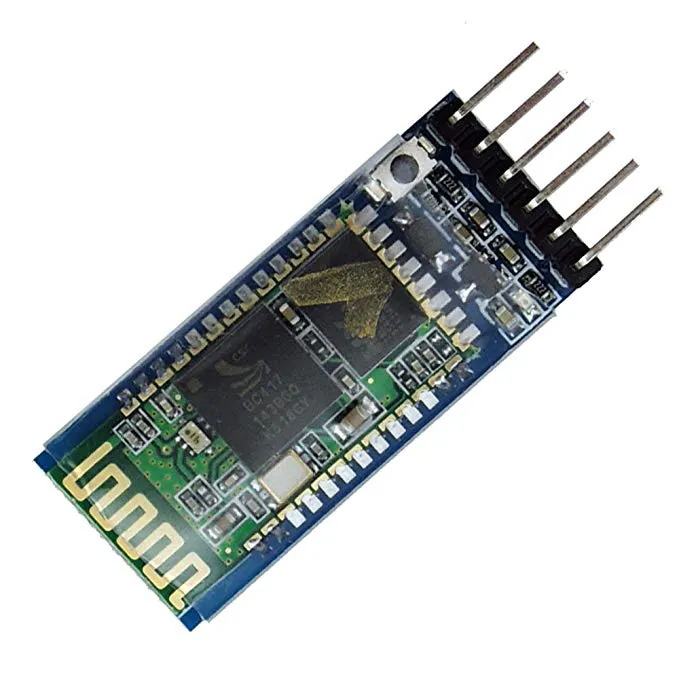HC-05 Bluetooth Module
×1
 Geared motor
×2
 Wheel
×2
 Caster wheel
×2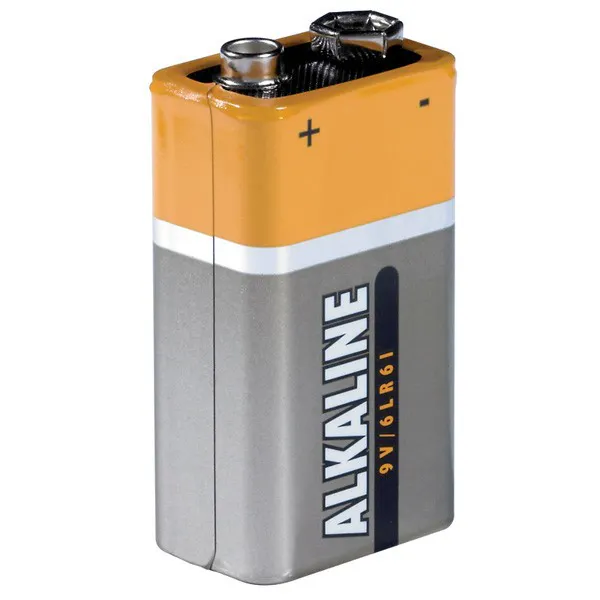9V battery (generic)
×3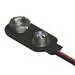9V Battery Clip
×3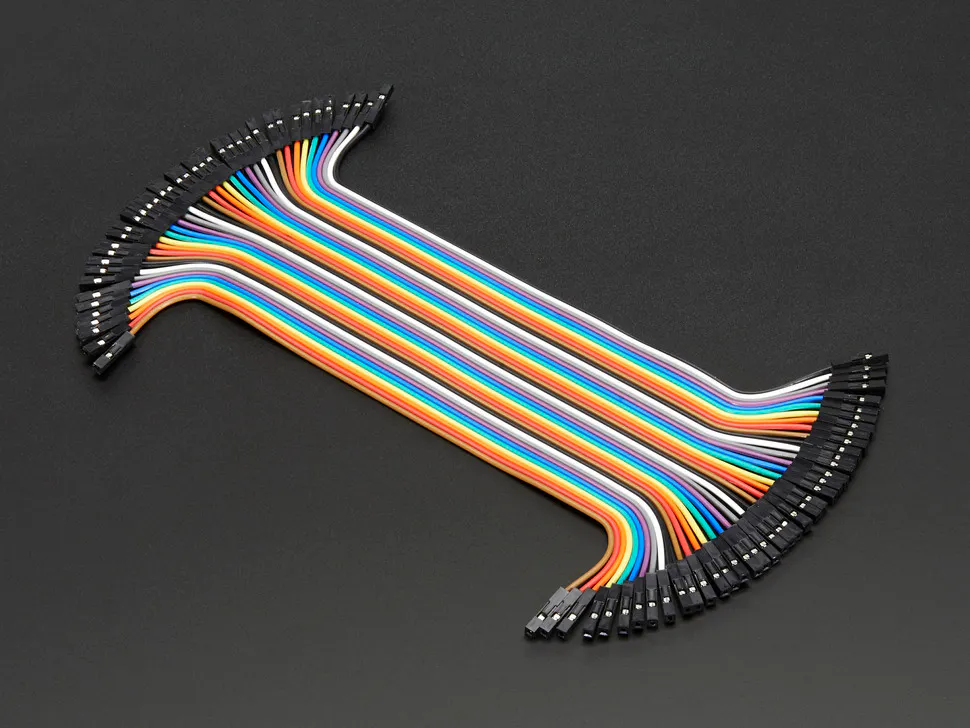Female/Female Jumper Wires
×8
 Plastic Box
×1

### Software apps and online services

 Bluetooth ElectronicsArduino IDE

### Hand tools and fabrication machinesSoldering iron (generic)Hot glue gun (generic)
 Screw driver

## Schematics

### Make your first Arduino robot schematic - Robotech maker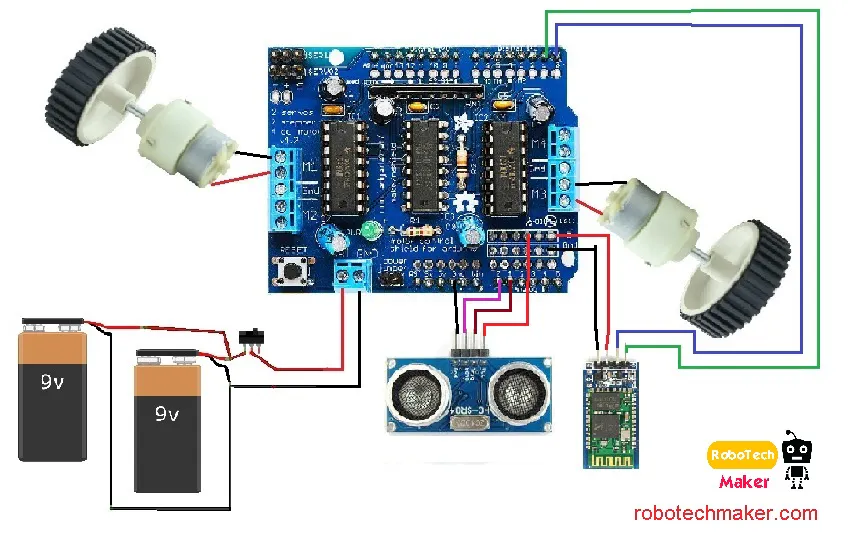## Code

### Bluetooth (Controlled Robot) Sketch

Arduino
```/*
Author:  Marcelo Moraes

This project contains public domain code.
The modification is allowed without notice.

*/

//Libraries
#include <AFMotor.h>

//Objects
AF_DCMotor motorRight(1, MOTOR12_64KHZ); // create motor #1, 64KHz pwm
AF_DCMotor motorLeft(3, MOTOR12_64KHZ);  // create motor #3, 64KHz pwm

//Constants and variable
char dataIn = 'S';
char determinant;
char det;
int vel = 0; //Bluetooth Stuff

void setup() {
Serial.begin(9600); // set up Serial library at 9600 bps

//Initalization messages
Serial.println("ArduinoBymyself - ROVERBot");
Serial.println("     AF Motor test!");

//turn off motors
motorRight.setSpeed(0);
motorLeft.setSpeed(0);
motorRight.run(RELEASE);
motorLeft.run(RELEASE);

}

void loop() {
det = check(); //call check() subrotine to get the serial code

//serial code analysis
switch (det){
case 'F': // F, move forward
motorRight.setSpeed(vel);
motorLeft.setSpeed(vel);
motorRight.run(FORWARD);
motorLeft.run(FORWARD);
det = check();
break;

case 'B': // B, move back
motorRight.setSpeed(vel);
motorLeft.setSpeed(vel);
motorRight.run(BACKWARD);
motorLeft.run(BACKWARD);
det = check();
break;

case 'L':// L, move wheels left
motorRight.setSpeed(vel);
motorLeft.setSpeed(vel/4);
motorRight.run(FORWARD);
motorLeft.run(FORWARD);
det = check();
break;

case 'R': // R, move wheels right
motorRight.setSpeed(vel/4);
motorLeft.setSpeed(vel);
motorRight.run(FORWARD);
motorLeft.run(FORWARD);
det = check();
break;

case 'I': // I, turn right forward
motorRight.setSpeed(vel/2);
motorLeft.setSpeed(vel);
motorRight.run(FORWARD);
motorLeft.run(FORWARD);
det = check();
break;

case 'J': // J, turn right back
motorRight.setSpeed(vel/2);
motorLeft.setSpeed(vel);
motorRight.run(BACKWARD);
motorLeft.run(BACKWARD);
det = check();
break;

case 'G': // G, turn left forward
motorRight.setSpeed(vel);
motorLeft.setSpeed(vel/2);
motorRight.run(FORWARD);
motorLeft.run(FORWARD);
det = check();
break;

case 'H': // H, turn left back
motorRight.setSpeed(vel);
motorLeft.setSpeed(vel/2);
motorRight.run(BACKWARD);
motorLeft.run(BACKWARD);
det = check();
break;

case 'S': // S, stop
motorRight.setSpeed(vel);
motorLeft.setSpeed(vel);
motorRight.run(RELEASE);
motorLeft.run(RELEASE);
det = check();
break;

}
}

//get bluetooth code received from serial port
int check(){
if (Serial.available() > 0){// if there is valid data in the serial port
dataIn = Serial.read();// stores data into a varialbe

//check the code
if (dataIn == 'F'){//Forward
determinant = 'F';
}
else if (dataIn == 'B'){//Backward
determinant = 'B';
}
else if (dataIn == 'L'){//Left
determinant = 'L';
}
else if (dataIn == 'R'){//Right
determinant = 'R';
}
else if (dataIn == 'I'){//Froward Right
determinant = 'I';
}
else if (dataIn == 'J'){//Backward Right
determinant = 'J';
}
else if (dataIn == 'G'){//Forward Left
determinant = 'G';
}
else if (dataIn == 'H'){//Backward Left
determinant = 'H';
}
else if (dataIn == 'S'){//Stop
determinant = 'S';
}
else if (dataIn == '0'){//Speed 0
vel = 0;
}
else if (dataIn == '1'){//Speed 25
vel = 25;
}
else if (dataIn == '2'){//Speed 50
vel = 50;
}
else if (dataIn == '3'){//Speed 75
vel = 75;
}
else if (dataIn == '4'){//Speed 100
vel = 100;
}
else if (dataIn == '5'){//Speed 125
vel = 125;
}
else if (dataIn == '6'){//Speed 150
vel = 150;
}
else if (dataIn == '7'){//Speed 175
vel = 175;
}
else if (dataIn == '8'){//Speed 200
vel = 200;
}
else if (dataIn == '9'){//Speed 225
vel = 225;
}
else if (dataIn == 'q'){//Speed 255
vel = 255;
}
else if (dataIn == 'U'){//Back Lights On
determinant = 'U';
}
else if (dataIn == 'u'){//Back Lights Off
determinant = 'u';
}
else if (dataIn == 'W'){//Front Lights On
determinant = 'W';
}
else if (dataIn == 'w'){//Front Lights Off
determinant = 'w';
}
else if (dataIn == 'V'){//Horn On
determinant = 'V';
}
else if (dataIn == 'v'){//Horn Off
determinant = 'v';
}
else if (dataIn == 'X'){//Extra On
determinant = 'X';
}
else if (dataIn == 'x'){//Extra Off
determinant = 'x';
}
}
return determinant;
}
```

### Final Sketch (Final Code)

Arduino
```/*
Author: Muhammed Azhar
visit - robotechmaker.com
*/

#include <AFMotor.h>
#define trigPin 14 // define the pins of your sensor
#define echoPin 15

//Objects
AF_DCMotor motorRight(1, MOTOR12_64KHZ); // create motor #1, 64KHz pwm
AF_DCMotor motorLeft(3, MOTOR12_64KHZ);  // create motor #3, 64KHz pwm

//Constants and variable
char dataIn = 'S';
char determinant;
char det;
int vel = 255; //Bluetooth Stuff

void setup() {
Serial.begin(9600); // set up Serial library at 9600 bps

pinMode(trigPin, OUTPUT);// set the trig pin to output (Send sound waves)
pinMode(echoPin, INPUT);// set the echo pin to input (recieve sound waves)

//Initalization messages
Serial.println(" Mr.robot");
Serial.println(" Reday for working!");

//turn off motors
motorRight.setSpeed(0);
motorLeft.setSpeed(0);
motorRight.run(RELEASE);
motorLeft.run(RELEASE);
}

void loop() {
det = check(); //call check() subrotine to get the serial code
//serial code analysis
switch (det){
case 'F': // F, move forward
motorRight.setSpeed(vel);
motorLeft.setSpeed(vel);
motorRight.run(FORWARD);
motorLeft.run(FORWARD);
det = check();
break;

case 'B': // B, move back
motorRight.setSpeed(vel);
motorLeft.setSpeed(vel);
motorRight.run(BACKWARD);
motorLeft.run(BACKWARD);
det = check();
break;

case 'L':// L, move wheels left
motorRight.setSpeed(vel);
motorLeft.setSpeed(vel/4);
motorRight.run(FORWARD);
motorLeft.run(FORWARD);
det = check();
break;

case 'R': // R, move wheels right
motorRight.setSpeed(vel/4);
motorLeft.setSpeed(vel);
motorRight.run(FORWARD);
motorLeft.run(FORWARD);
det = check();
break;

case 'I': // I, turn right forward
motorRight.setSpeed(vel/2);
motorLeft.setSpeed(vel);
motorRight.run(FORWARD);
motorLeft.run(FORWARD);
det = check();
break;

case 'J': // J, turn right back
motorRight.setSpeed(vel/2);
motorLeft.setSpeed(vel);
motorRight.run(BACKWARD);
motorLeft.run(BACKWARD);
det = check();
break;

case 'G': // G, turn left forward
motorRight.setSpeed(vel);
motorLeft.setSpeed(vel/2);
motorRight.run(FORWARD);
motorLeft.run(FORWARD);
det = check();
break;

case 'H': // H, turn left back
motorRight.setSpeed(vel);
motorLeft.setSpeed(vel/2);
motorRight.run(BACKWARD);
motorLeft.run(BACKWARD);
det = check();
break;

case 'S':
// S, stop
motorRight.setSpeed(vel);
motorLeft.setSpeed(vel);
motorRight.run(RELEASE);
motorLeft.run(RELEASE);
det = check();
break;

case 'm':

//for wall follower robot.
motorRight.setSpeed(vel); //set the speed of the motors, between 0-255
motorLeft.setSpeed (vel);

long duration, distance; // start the scan
digitalWrite(trigPin, LOW);
delayMicroseconds(2); // delays are required for a succesful sensor operation.
digitalWrite(trigPin, HIGH);

delayMicroseconds(10); //this delay is required as well!
digitalWrite(trigPin, LOW);
duration = pulseIn(echoPin, HIGH);
distance = (duration/2) / 29.1;// convert the distance to centimeters.
if (distance < 30)/*The distance that need to to keep with the wall */ {
Serial.println ("Wall is ditected!" );
Serial.println (" Started following the wall ");
Serial.println (" Turning !");
motorRight.setSpeed(vel);
motorLeft.setSpeed(0);
motorRight.run(FORWARD);
motorLeft.run(RELEASE);
delay(500); // wait for a second
}

else {
Serial.println ("No Wall detected. turning round");
delay (15);
motorRight.setSpeed(0);
motorLeft.setSpeed(vel);
motorRight.run(RELEASE);
motorLeft.run (FORWARD);

}
break;

case 'b':
//obstacle avoider robot

motorRight.setSpeed(vel); //set the speed of the motors, between 0-255
motorLeft.setSpeed (vel);

digitalWrite(trigPin, LOW);
delayMicroseconds(2); // delays are required for a succesful sensor operation.
digitalWrite(trigPin, HIGH);

delayMicroseconds(10); //this delay is required as well!
digitalWrite(trigPin, LOW);
if (Adistance < 25)/*if there's an obstacle 25 centimers, ahead, do the following: */ {
Serial.println ("Close Obstacle detected!" );
Serial.println ("Obstacle Details:");
Serial.print ("Distance From Robot is " );
Serial.print ( " CM!");// print out the distance in centimeters.

Serial.println (" The obstacle is declared a threat due to close distance. ");
Serial.println (" Turning !");
motorRight.setSpeed(vel);
motorLeft.setSpeed(vel);
motorLeft.run(BACKWARD);  // Turn as long as there's an obstacle ahead.
motorRight.run (FORWARD);

}
else {
Serial.println ("No obstacle detected. going forward");
delay (15);
motorRight.setSpeed(vel);
motorLeft.setSpeed(vel);
motorRight.run(FORWARD); //if there's no obstacle ahead, Go Forward!
motorLeft.run(FORWARD);

}
break;
}
}

//get bluetooth code received from serial port
int check(){
if (Serial.available() > 0){// if there is valid data in the serial port
dataIn = Serial.read();// stores data into a varialbe

//check the code
if (dataIn == 'F'){//Forward
determinant = 'F';
}
else if (dataIn == 'B'){//Backward
determinant = 'B';
}
else if (dataIn == 'L'){//Left
determinant = 'L';
}
else if (dataIn == 'R'){//Right
determinant = 'R';
}
else if (dataIn == 'I'){//Froward Right
determinant = 'I';
}
else if (dataIn == 'J'){//Backward Right
determinant = 'J';
}
else if (dataIn == 'G'){//Forward Left
determinant = 'G';
}
else if (dataIn == 'H'){//Backward Left
determinant = 'H';
}
else if (dataIn == 'S'){//Stop
determinant = 'S';
}
else if (dataIn == '0'){//Speed 0
vel = 0;
}
else if (dataIn == '1'){//Speed 25
vel = 25;
}
else if (dataIn == '2'){//Speed 50
vel = 50;
}
else if (dataIn == '3'){//Speed 75
vel = 75;
}
else if (dataIn == '4'){//Speed 100
vel = 100;
}
else if (dataIn == '5'){//Speed 125
vel = 125;
}
else if (dataIn == '6'){//Speed 150
vel = 150;
}
else if (dataIn == '7'){//Speed 175
vel = 175;
}
else if (dataIn == '8'){//Speed 200
vel = 200;
}
else if (dataIn == '9'){//Speed 225
vel = 225;
}
else if (dataIn == 'b'){//Extra On
determinant = 'b';
}
else if (dataIn == 'm'){//Extra On
determinant = 'm';
}

}
return determinant;
}
```

### Obstacle Avoider Sketch

Arduino
```/*
Author: Muhammed Azhar
visit - robotechmaker.com
*/

#include <AFMotor.h>
#define trigPin 14 // define the pins of your sensor
#define echoPin 15

int vel = 255; // Speed of the robot

//Objects
AF_DCMotor motorRight(1, MOTOR12_64KHZ); // create motor #1, 64KHz pwm
AF_DCMotor motorLeft(3, MOTOR12_64KHZ);  // create motor #3, 64KHz pwm

void setup() {
Serial.begin(9600); // set up Serial library at 9600 bps

pinMode(trigPin, OUTPUT);// set the trig pin to output (Send sound waves)
pinMode(echoPin, INPUT);// set the echo pin to input (recieve sound waves)

//Initalization messages
Serial.println(" Mr.robot");
Serial.println(" Reday for working!");

//turn off motors
motorRight.setSpeed(0);
motorLeft.setSpeed(0);
motorRight.run(RELEASE);
motorLeft.run(RELEASE);
}

void loop() {
//obstacle avoider robot

motorRight.setSpeed(vel); //set the speed of the motors, between 0-255
motorLeft.setSpeed (vel);

long duration, distance; // start the scan
digitalWrite(trigPin, LOW);
delayMicroseconds(2); // delays are required for a succesful sensor operation.
digitalWrite(trigPin, HIGH);

delayMicroseconds(10); //this delay is required as well!
digitalWrite(trigPin, LOW);
duration = pulseIn(echoPin, HIGH);
distance = (duration/2) / 29.1;// convert the distance to centimeters.
if (distance < 25)/*if there's an obstacle 25 centimers, ahead, do the following: */ {
Serial.println ("Close Obstacle detected!" );
Serial.println ("Obstacle Details:");
Serial.print ("Distance From Robot is " );
Serial.print ( distance);
Serial.print ( " CM!");// print out the distance in centimeters.

Serial.println (" The obstacle is declared a threat due to close distance. ");
Serial.println (" Turning !");
motorRight.setSpeed(vel);
motorLeft.setSpeed(vel);
motorLeft.run(BACKWARD);  // Turn as long as there's an obstacle ahead.
motorRight.run (FORWARD);

}
else {
Serial.println ("No obstacle detected. going forward");
delay (15);
motorRight.setSpeed(vel);
motorLeft.setSpeed(vel);
motorRight.run(FORWARD); //if there's no obstacle ahead, Go Forward!
motorLeft.run(FORWARD);

}
}
```

### Wall Follower Sketch

Arduino
```/*
Author: Muhammed Azhar
visit - robotechmaker.com
*/

#include <AFMotor.h>
#define trigPin 14 // define the pins of your sensor
#define echoPin 15

int vel = 255; // Speed of the robot

//Objects
AF_DCMotor motorRight(1, MOTOR12_64KHZ); // create motor #1, 64KHz pwm
AF_DCMotor motorLeft(3, MOTOR12_64KHZ);  // create motor #3, 64KHz pwm

void setup()
{
Serial.begin(9600); // set up Serial library at 9600 bps

pinMode(trigPin, OUTPUT);// set the trig pin to output (Send sound waves)
pinMode(echoPin, INPUT);// set the echo pin to input (recieve sound waves)

//Initalization messages
Serial.println(" Mr.robot");
Serial.println(" Reday for working!");

//turn off motors
motorRight.setSpeed(0);
motorLeft.setSpeed(0);
motorRight.run(RELEASE);
motorLeft.run(RELEASE);
}

void loop()
{

//for wall follower robot.
motorRight.setSpeed(vel); //set the speed of the motors, between 0-255
motorLeft.setSpeed(vel);

long duration, distance; // start the scan
digitalWrite(trigPin, LOW);
delayMicroseconds(2); // delays are required for a succesful sensor operation.
digitalWrite(trigPin, HIGH);

delayMicroseconds(10); //this delay is required as well!
digitalWrite(trigPin, LOW);
duration = pulseIn(echoPin, HIGH);
distance = (duration / 2) / 29.1;// convert the distance to centimeters.
if (distance < 30)/*The distance that need to to keep with the wall */
{
Serial.println("Wall is ditected!");
Serial.println(" Started following the wall ");
Serial.println(" Turning !");
motorRight.setSpeed(vel);
motorLeft.setSpeed(0);
motorRight.run(FORWARD);
motorLeft.run(RELEASE);
delay(500); // wait for a second
}

else
{
Serial.println("No Wall detected. turning round");
delay(15);
motorRight.setSpeed(0);
motorLeft.setSpeed(vel);
motorRight.run(RELEASE);
motorLeft.run(FORWARD);
}
}
```

## Credits

### Muhammed Azhar

1 project • 108 followers
I am Muhammed Azhar a 17 y/o maker from India. Visit my blog for more projects- robotechmaker.com